Samacheer Kalvi 8th Maths Solutions Term 1 Chapter 3 Algebra Ex 3.2

Students can Download Maths Chapter 3 Algebra Ex 3.2 Questions and Answers, Notes Pdf, Samacheer Kalvi 8th Maths Book Solutions Guide Pdf helps you to revise the complete Tamilnadu State Board New Syllabus and score more marks in your examinations.Tamilnadu Samacheer Kalvi 8th Maths Solutions Term 1 Chapter 3 Algebra Ex 3.2

Question 1.
Fill in the blanks: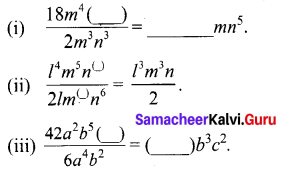Solution:
(i) $$\frac{18 m^{4}\left(n^{8}\right)}{2 m^{(3)} n^{3}}$$ = 9 mn5
(ii) $$\frac{l^{4} m^{5} n^{(7)}}{2 l m^{(3)} n^{6}}=\frac{l^{3} m^{2} n}{2}$$
(iii) $$\frac{42 a^{4} b^{5}\left(c^{2}\right)}{6(a)^{4}(b)^{2}}$$ = (7)b3c2

Question 2.
Say True or False:
(i) 5x3y ÷ 4x2 = 2xy
(ii) 7ab2 ÷ 14ab = 2b2
Solution:
(i) True
(ii) False

Question 3.
(i) 27y3 ÷ 3y
(ii) x3y2 ÷ x2y
(iii) 45x3y2z4 ÷ (-15xyz)
(iv) (3xy)2 ÷ 9xy
Solution: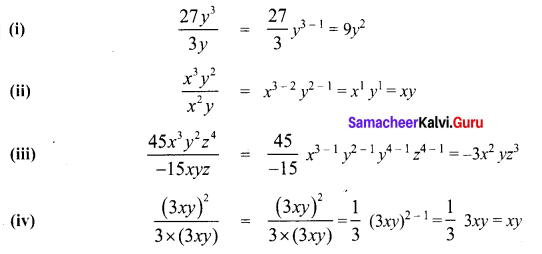Question 4.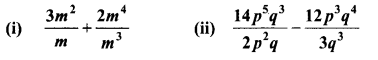Solution: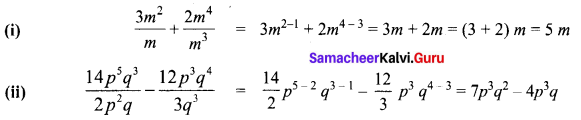Question 5.
Divide
(i) 32y2 – 8yz by 2y
(ii) (4m2 n3 + 16m4 n2 – mn) by 2 mn
(iii) 10 (4x – 8y) by 5 (x – 2y)
(iv) 81 (94q2r3 + 2p3q3r2 – 5p2q2r2) by (3pqr)2
Solution: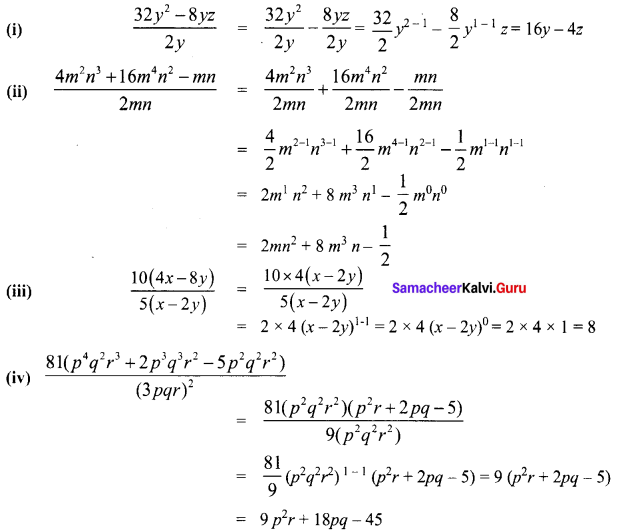Question 6.
Find Adirai’s percentage of marks who scored 25m3n2p out of 100m2np
Solution:
Total marks = 100 m2np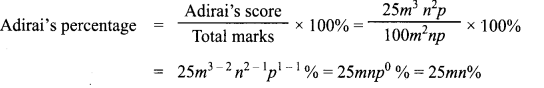Question 7.
Identify the error and correct them.
(i) 7y2 – y2 + 3y2 = 10y2
(ii) 6xy + 3xy = 9x2y2
(iii) m (4m – 3) = 4m2 – 3
(iv) (4n)2 – 2n + 3 = 4n2 – 2n + 3
(v) (x – 2) (x + 3) = x2 – 6
Solution:
(i) 7y2 – y2 + 3y2 = (7 – 1 + 3)y2 = (6 + 3)y2 = 9y2
(ii) 6xy + 3xy = (6 + 3)xy = 9xy
(iii) m (4m – 3) = m (4m) + m (-3) = 4m2 – 3m
(iv) (4n)2 – 2n + 3 = 16n2 – 2n + 3
(v) (x – 2) (x + 3) = x (x + 3) – 2 (x + 3) = x (x) + (x) × 3 + (-2) (x) + (-2) (3)
= x2 + 3x – 2x – 6 = x2 + x – 6